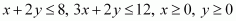# Linear Programming Chapter 12 Class 12 Maths NCERT Textbook PDF

NCERT Solutions for Class 12 Maths Chapter 12‘ PDF Quick download link is given at the bottom of this article. You can see the PDF demo, size of the PDF, page numbers, and direct download Free PDF of ‘Ncert Class 12 Maths Chapter 12 Exercise Solution’ using the download button.

### Chapter 12: Linear Programming

#### 12.1 Introduction

In earlier classes, we have discussed systems of linear equations and their applications in day-to-day problems.

In Class XI, we have studied linear inequalities and systems of linear inequalities in two variables and their solutions by graphical method.

Many applications in mathematics involve systems of inequalities/equations. In this chapter, we shall apply the systems of linear inequalities/equations to solve some real-life problems of the type given below:

A furniture dealer deals in only two items–tables and chairs. He has Rs 50,000 to invest and has storage space of at most 60 pieces.

A table costs Rs 2500 and a chair Rs 500. He estimates that from the sale of one table, he can make a profit of Rs 250 and that from the sale of one chair a profit of Rs 75.

He wants to know how many tables and chairs he should buy with the available money so as to maximize his total profit, assuming that he can sell all the items which he buys.

Such types of problems which seek to maximize (or, minimize) profit (or, cost) form a general class of problems called optimization problems.

Thus, an optimization of the problem may involve finding maximum profit, minimum cost, minimum use of resources, etc.

A special but very important class of optimization problems is linear programming problems. The above-stated optimization problem is an example of a linear programming problem.

Linear programming problems are of much interest because of their wide applicability in industry, commerce, management science, etc.

In this chapter, we shall study some linear programming problems and their solutions by graphical method only, though there are many other methods also to solve such problems.

### NCERT Solutions Class 12 Maths Chapter 12 Linear Programming

1. Maximise Z = 3x + 4y

Subject to the constraints:

Solution:

The feasible region determined by the constraints, x + y ≤ 4, x ≥ 0, y ≥ 0, is given below

O (0, 0), A (4, 0), and B (0, 4) are the corner points of the feasible region. The values of Z at these points are given below

Hence, the maximum value of Z is 16 at the point B (0, 4)

2. Minimise Z = −3x + 4y

subject to.

Solution:

The feasible region is determined by the system of constraints,is given below

O (0, 0), A (4, 0), B (2, 3) and C (0, 4) are the corner points of the feasible region

The values of Z at these corner points are given below

Hence, the minimum value of Z is – 12 at the point (4, 0)

3. Maximise Z = 5x + 3y

subject to.

Solution:

The feasible region determined by the system of constraints, 3x + 5y ≤ 15, 5x + 2y ≤ 10, x ≥ 0, and y ≥ 0, are given below

O (0, 0), A (2, 0), B (0, 3) and C (20 / 19, 45 / 19) are the corner points of the feasible region. The values of Z at these corner points are given below

Hence, the maximum value of Z is 235 / 19 at the point (20 / 19, 45 / 19)

4. Minimise Z = 3x + 5y

such that.

Solution:

The feasible region determined by the system of constraints, x + 3y ≥ 3, x + y ≥ 2, and x, y ≥ 0 is given below

It can be seen that the feasible region is unbounded.

The corner points of the feasible region are A (3, 0), B (3 / 2, 1 / 2), and C (0, 2)

The values of Z at these corner points are given below

7 may or may not be the minimum value of Z because the feasible region is unbounded

For this purpose, we draw the graph of the inequality, 3x + 5y < 7, and check the resulting half-plane has common points with the feasible region or not

Hence, it can be seen that the feasible region has no common point with 3x + 5y < 7

Thus, the minimum value of Z is 7 at point B (3 / 2, 1 / 2)

5. Maximise Z = 3x + 2y

subject to.

Solution:

The feasible region determined by the constraints, x + 2y ≤ 10, 3x + ≤ 15, x ≥ 0, and y ≥ 0, is given below

A (5, 0), B (4, 3), C (0, 5) and D (0, 0) are the corner points of the feasible region.

The values of Z at these corner points are given below

Hence, the maximum value of Z is 18 at the point (4, 3)